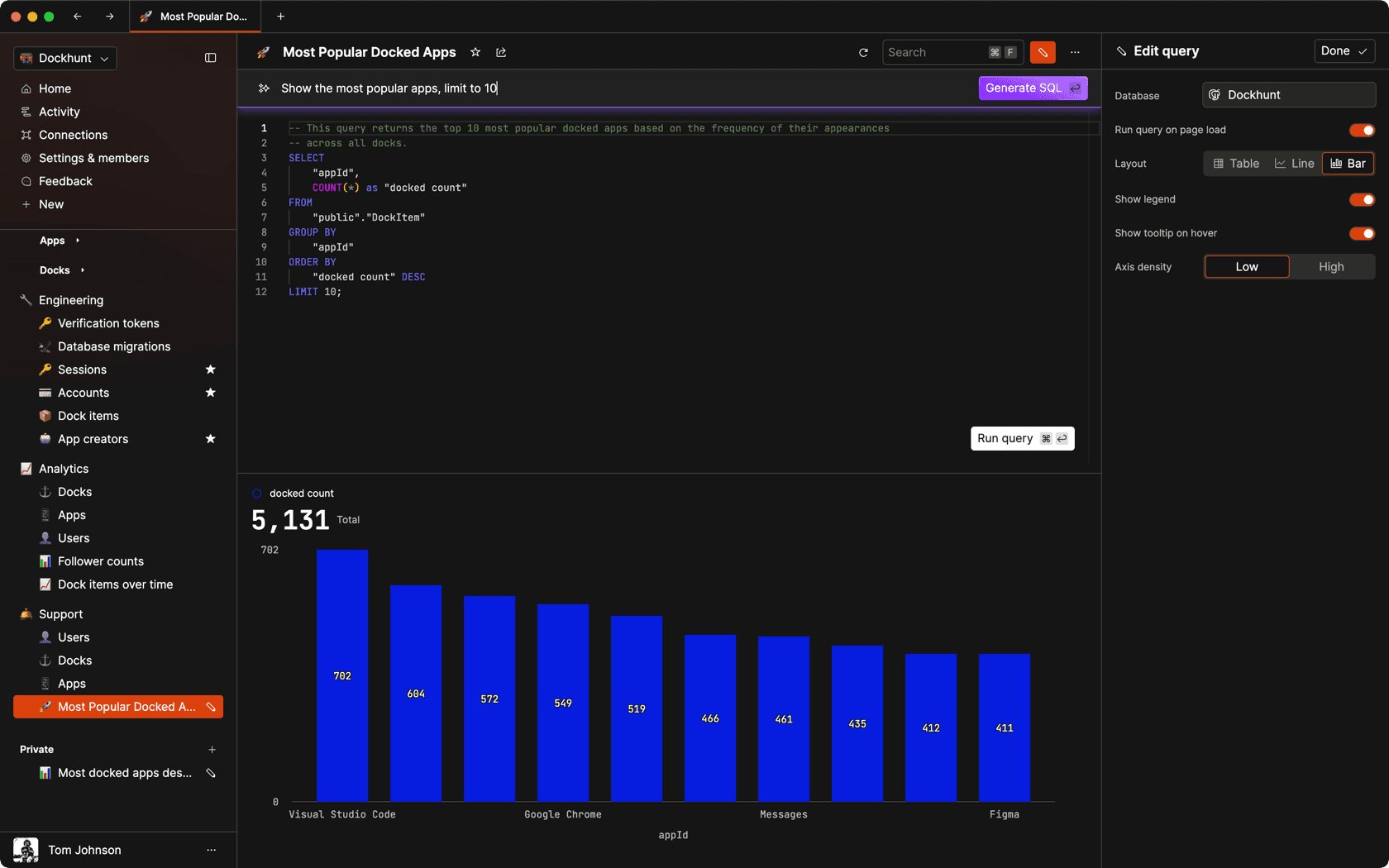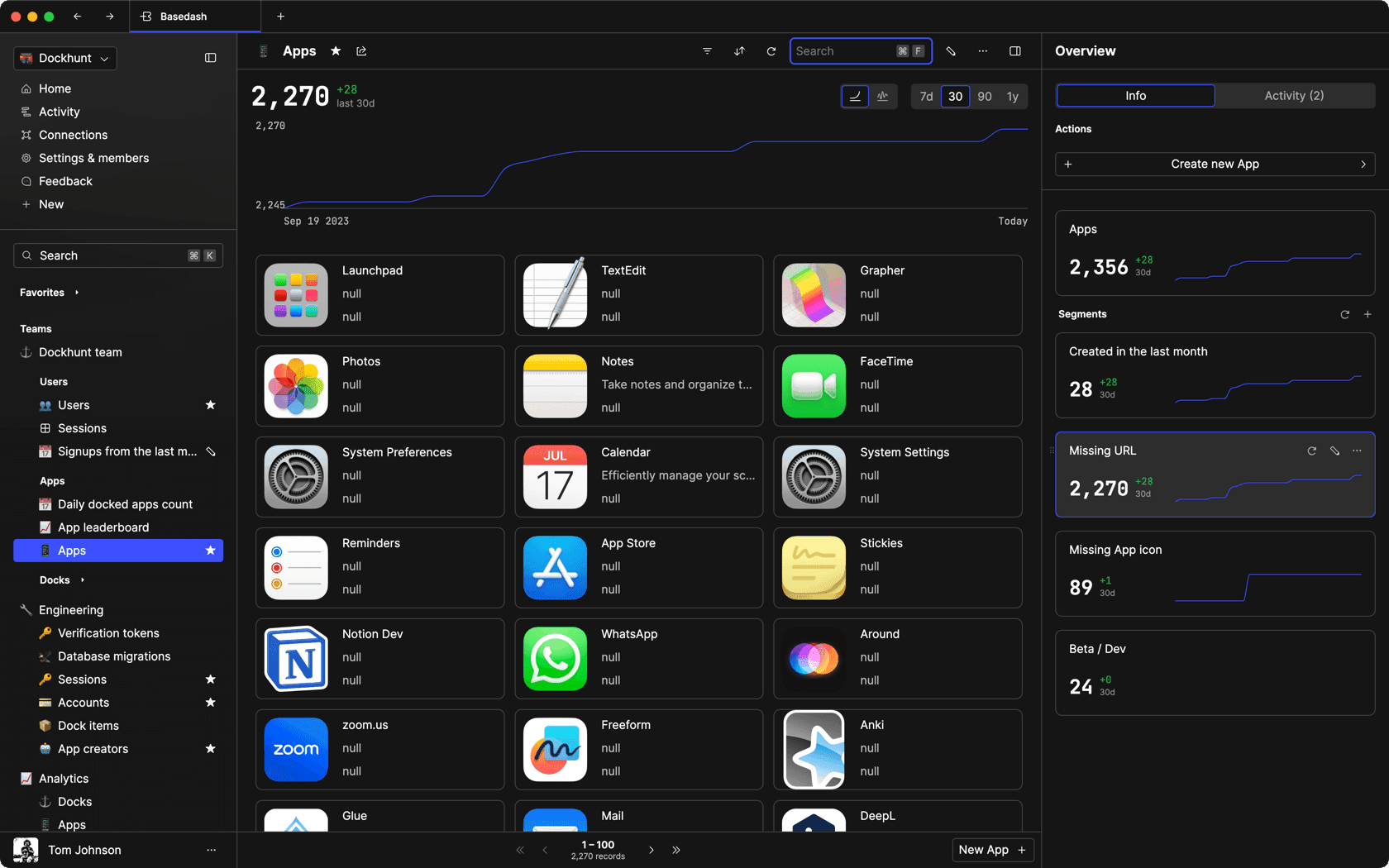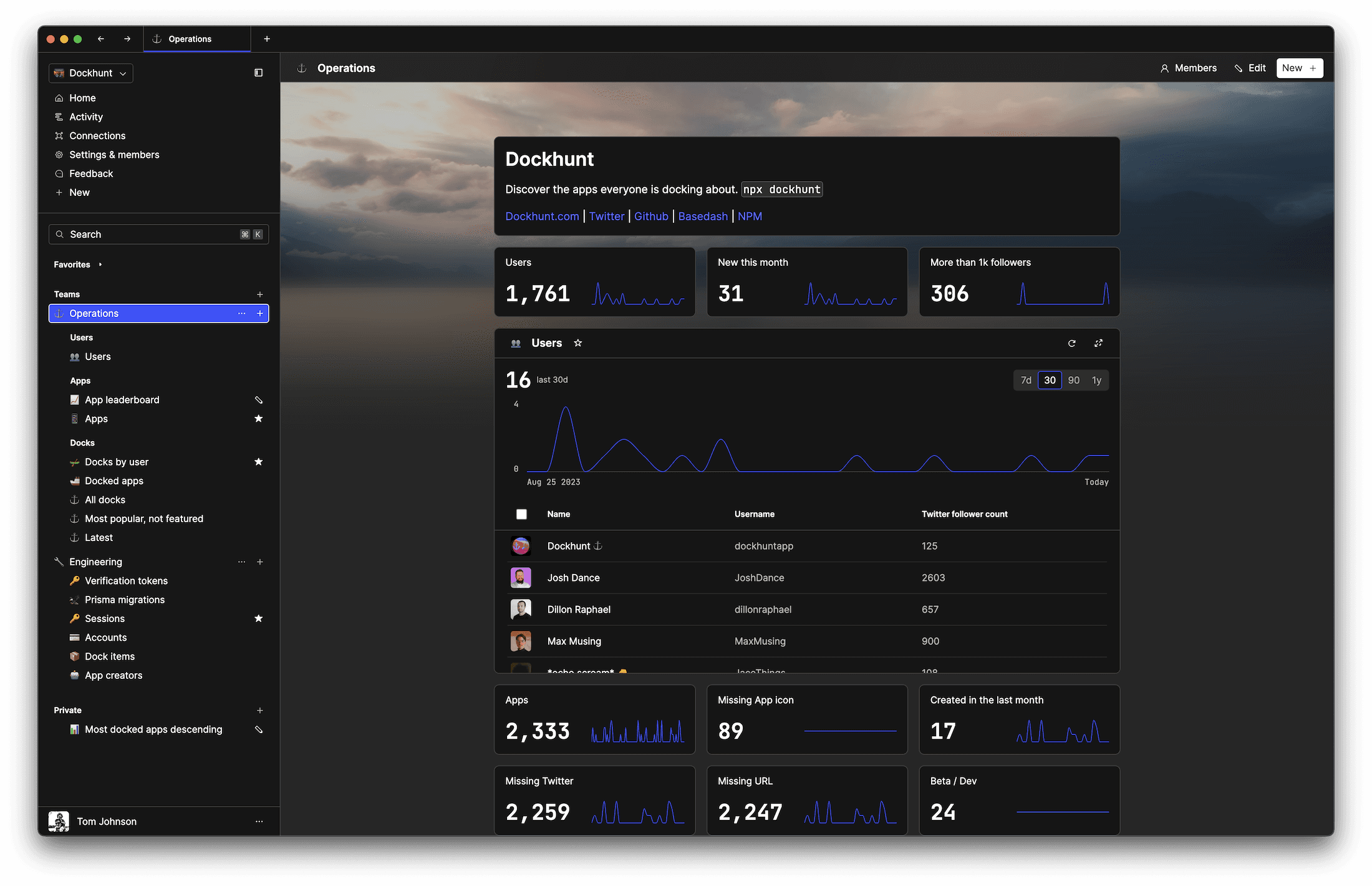# How to declare a multidimensional array in JavaScriptself.__wrap_n=self.__wrap_n||(self.CSS&&CSS.supports("text-wrap","balance")?1:2);self.__wrap_b=(e,r,t)=>{t=t||document.querySelector(`[data-br="\${e}"]`);let n=t.parentElement,a=s=>t.style.maxWidth=s+"px";t.style.maxWidth="";let o,i=n.clientWidth,l=n.clientHeight,c=i/2-.25,f=i+.5;if(i){for(a(c),c=Math.max(t.scrollWidth,c);c+1<f;)o=Math.round((c+f)/2),a(o),n.clientHeight===l?f=o:c=o;a(f*r+i*(1-r))}t.__wrap_o||typeof ResizeObserver<"u"&&(t.__wrap_o=new ResizeObserver(()=>{self.__wrap_b(0,+t.dataset.brr,t)})).observe(n)};self.__wrap_n!=1&&self.__wrap_b(":Rj0pm:",1)

November 4, 2023

Multidimensional arrays in JavaScript are arrays whose elements are also arrays. These can be used to represent complex data structures like matrices, grids, or any other nested data configuration.

## Understanding multidimensional arrays

Before delving into the syntax, it's crucial to understand that JavaScript does not have true multidimensional arrays like some other languages. Instead, we create an array of arrays. Each element of the main array (first dimension) is another array (second dimension), and this can continue for as many dimensions as needed.

## Declaring a two-dimensional array

The simplest form of a multidimensional array is a two-dimensional array. It can represent a table or grid-like structure.

### With array literals

Here's how to declare a two-dimensional array using array literals:

```let twoDimensionalArray = [
[1, 2, 3],
[4, 5, 6],
[7, 8, 9]
];```

Each inner array represents a row in the two-dimensional array.

### Using a constructor

Alternatively, you can use the `Array` constructor to declare an empty two-dimensional array and fill it later:

```let rows = 3;
let cols = 3;
let twoDimensionalArray = new Array(rows);

for (let i = 0; i < rows; i++) {
twoDimensionalArray[i] = new Array(cols);
}```

## Declaring higher-dimensional arrays

For arrays with more than two dimensions, the concept is the same, but you add more levels of nesting.

### Three-dimensional array example

Here's an example of declaring a three-dimensional array with array literals:

```let threeDimensionalArray = [
[
[1, 2, 3],
[4, 5, 6],
[7, 8, 9]
],
[
[10, 11, 12],
[13, 14, 15],
[16, 17, 18]
],
[
[19, 20, 21],
[22, 23, 24],
[25, 26, 27]
]
];```

### Initializing higher dimensions dynamically

Creating higher-dimensional arrays dynamically can involve nested loops:

```let depth = 3;
let rows = 3;
let cols = 3;
let threeDimensionalArray = new Array(depth);

for (let i = 0; i < depth; i++) {
threeDimensionalArray[i] = new Array(rows);
for (let j = 0; j < rows; j++) {
threeDimensionalArray[i][j] = new Array(cols);
}
}```

## Initializing with functions

For complex or large multidimensional arrays, initialization functions can help manage the complexity.

### Function to create two-dimensional array

```function create2DArray(rows, cols) {
let array = new Array(rows);

for (let i = 0; i < rows; i++) {
array[i] = new Array(cols);
}

return array;
}

let myArray = create2DArray(3, 3);```

### Function to create any dimension array

For a more generic solution:

```function createMultiDimensionalArray(lengths) {
function createArray(lengths) {
if (lengths.length === 0) {
return undefined;
}

let length = lengths;
let arr = new Array(length);

for (let i = 0; i < length; i++) {
arr[i] = createArray(lengths.slice(1));
}

return arr;
}

return createArray(lengths);
}

let myArray = createMultiDimensionalArray([3, 3, 3]);```

## Filling multidimensional arrays

Once declared, you can fill multidimensional arrays using loops:

```for (let i = 0; i < threeDimensionalArray.length; i++) {
for (let j = 0; j < threeDimensionalArray[i].length; j++) {
for (let k = 0; k < threeDimensionalArray[i][j].length; k++) {
threeDimensionalArray[i][j][k] = i + j + k;
}
}
}```

## Tips for working with multidimensional arrays

• Consistency: Ensure all nested arrays at the same level have the same length to avoid irregular data structures.
• Access: Use multiple index brackets to access elements (e.g., `myArray`).
• Higher dimensions: Keep track of the dimensions as you nest arrays. It's easy to get lost in the brackets with more than three dimensions.
• Memory: Large multidimensional arrays can consume significant memory. Initialize only what you need.

JavaScript's flexible array structures allow for easy creation and manipulation of multidimensional arrays, as long as you keep track of the dimensions and properly manage the nested structures.

# Ship faster, worry less with Basedash

You're busy enough with product work to be weighed down building, maintaining, scoping and developing internal apps and admin panels. Forget all of that, and give your team the admin panel that you don't have to build. Launch in less time than it takes to run a standup.

You're busy enough with product work to be weighed down building, maintaining, scoping and developing internal apps and admin panels. Forget all of that, and give your team the admin panel that you don't have to build. Launch in less time than it takes to run a standup.

You're busy enough with product work to be weighed down building, maintaining, scoping and developing internal apps and admin panels. Forget all of that, and give your team the admin panel that you don't have to build. Launch in less time than it takes to run a standup.### Dashboards and charts

Effortlessly make charts and create a space for your team to work together towards shared goals and metrics.

### SQL composer with AISOC II
Type 2 Certified

### Features

Proudly carbon-neutral.SOC II
Type 2 Certified

### Features

Proudly carbon-neutral.SOC II
Type 2 Certified